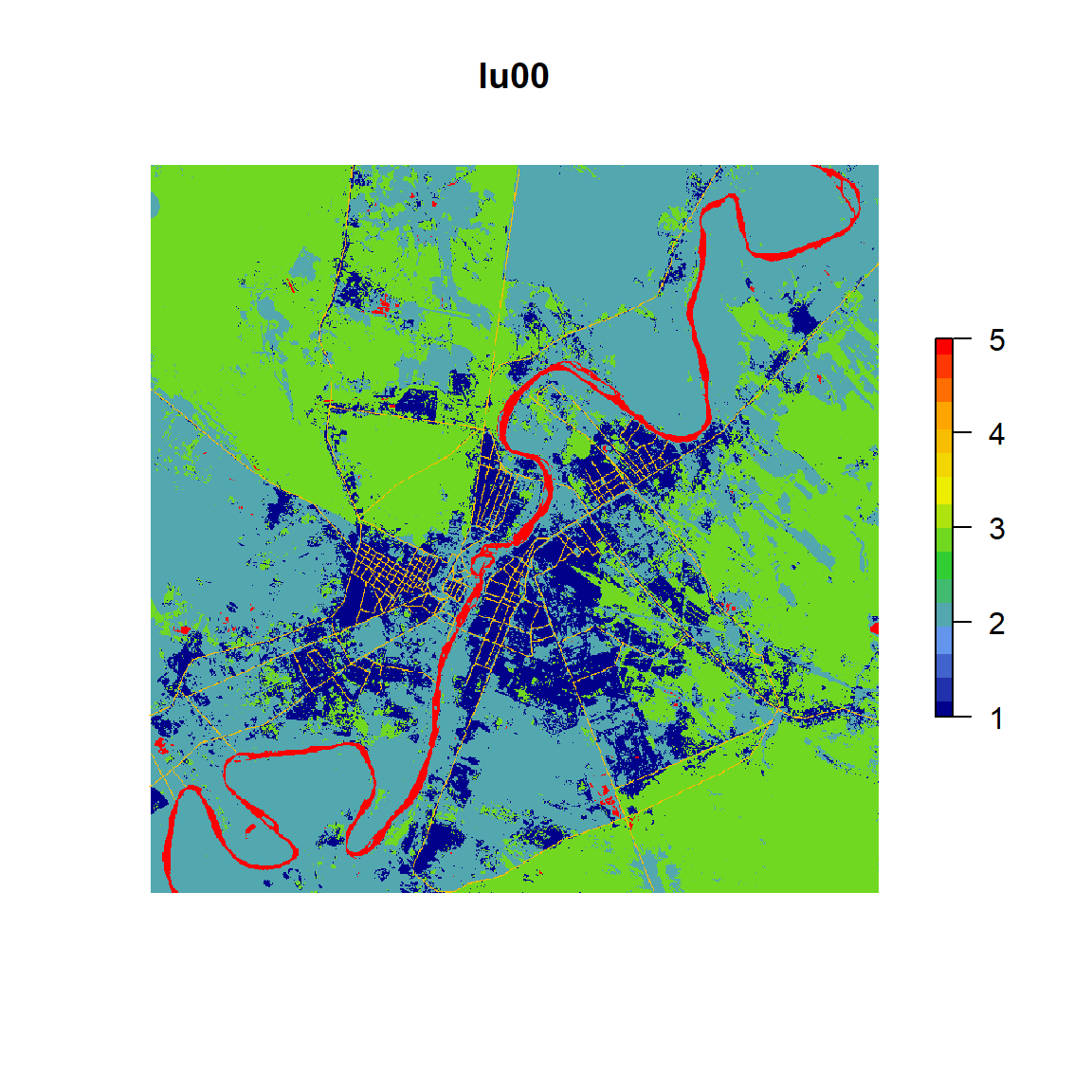DoTRules - Dictionary of Trusted Rules for modelling land-use transition potential.

This document illustrates the stepwise procedure of transition potential mapping using DoTRules. Please follow the below workflow for implementing the DoTRules algorithm in R. The R scripts for each footstep of the whole implementation procedure may be viewed by clicking on code buttons. The algorithm consists of 6 steps:

1. Assembling the data
2. Calculation of Shannon Entropy
3. Creating a rule set
4. Handling of NULL values
5. Calculating the uncertainty of land-use prediction
6. Classification of land-use according to the dictionary

## Step1: Assembling the data

### Reading the files

In this example the data was assembled from a set of .tif files. The .tif dataset is fully discussed in the manuscript (please see \(Table 1\)). To download the dataset click HERE. The applied variables or criteria for implementation of transition potential maps include three groups of predictor variables \(J =[j_1, j_2, ., j_n]\) and one response variable such as below:

1. Predictor variables:
• Cell state: that is land-use map of 1985 (lu85).
• Neighbourhood variables: including neighbouring urban (N8nnU), agriculture (N8nnA), bare lands (N8nnB), road (N8nnR) and water (N8nnW) cells within a 3 by 3 neighbourhood kernal.
• Suitability variables: including distance from road (disRoad), distance from drainage networks (disDrn), slope angle (slope) and distance from urban edge (disUrban).
1. Response variable:
• That is land-use map of 2000 (lu00).
``````# Setting up working directory
setwd(dirname(rstudioapi::getActiveDocumentContext()\$path))

# Importing the files from local drive
J.names <- c("lu85", "N8nnW", "N8nnR",  "N8nnB",  "N8nnA",  "N8nnU", "disRoad", "disDrn", "slope",  "disUrban")
y.names <- "lu00"
J <- file.path(getwd(), paste0(J.names, ".tif"))
y <- file.path(getwd(), paste0(y.names, ".tif"))

# Setting up data variables including each j in J as well as y
dat  <- Map(raster::raster, c(y, J))

# Settins up file names
names(dat) <- c(y.names, J.names)

# Save dimensions for later use
dims <- c(nrow=nrow(dat[]), ncol=ncol(dat[]))``````

Each file had 846 rows and 868 columns. We used one file \(y=\)[lu00] as a response variable and others: \(J=\)[lu85, N8nnW, N8nnR, N8nnB, N8nnA, N8nnU, disRoad, disDrn, slope, disUrban] as predictors.

Below is our response variable:

``````# Main function for plotting variables
plotRaster <- function(rst, ...) {
cols <- c("darkblue", "cornflowerblue", "limegreen", "yellow2", "orange", "red")
rst <- raster::as.matrix(rst)
rst <- raster::raster(rst)
raster::plot(rst, col=colorRampPalette(cols)(16), axes=FALSE, bty='n', box=FALSE, ...)
}

plotRaster(dat[], main=names(dat))``````The predictor variables are also demonstrated here:

``````par(mfrow=c(2,3))

for(i in 2:11) {
plotRaster(dat[[i]], main=names(dat)[i])
}``````